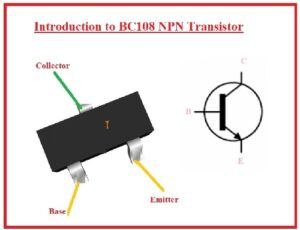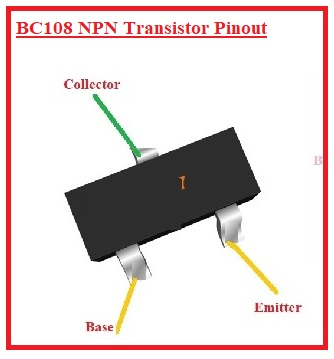Hello, electronic students welcome to new post. We will discuss Introduction to BC108 NPN Transistor. The transistor is a general-purpose electronic component used in different projects and circuits as amplifiers and switches. It comes with three pinouts EMitter, base, and collector. Transistor has two configurations NPN and PNP. In the PNP transistor, there are two P regions doped with hole carriers; in N, electrons are charge carriers.

Potential barriers exist in the transistor that is crossed by the holes and electrons to make the conduction. In this post we will discuss BC108 NPN Transistor pinouts, working and applications.

## Introduction to BC108 NPN Transistor

• BC108 transistor is a semiconductor module that is mostly used in such circuits where amplification is needed as well as power amplification and is employed as a switch.
• It is NPN configured transistor and uses a small quantity of input supply volts are the base so the needed current passes in the transistor.
• Before use of this transistor in amplification circuits must check the gain value from the datasheet of the transistor.
• Since the different value of gain is given by the different manufacturers must note before the application of these components.
• The highest value of collector current for this transistor is two hundred milliamperes and VBE is five volts.
• Since this transistor, if NPN is configured so there are two N regions and one P region.### BC108 NPN Transistor Pinout

• There is three pinouts this transistor has.
• EMitter: current goes from this part to collector
• Base: It works as a control for transistor
• Collector: It is larger part of the components and has larger current### BC108 Features

• Different features of this transistor are mentioned here
• Its operating temperature is minus sixty-five to one seventy-five
• The transition frequency value is one fifty megahertz
• The value of collector dissipation is 0.3 watts
• VCB is thirty volts
• It comes in TO-18 packages
• The Base current is ten millimperes
• VBE is five volt
• IC is two hundred milliamperes
• The current gain is nine hundred
• It is NPN configured transistor

### BC108 Applications

• Different applications of this transistor are explained here
• It used in radio frequency circuits
• Switching circuits
• Power amplifiers
• LED dimmers
• Used in flashers